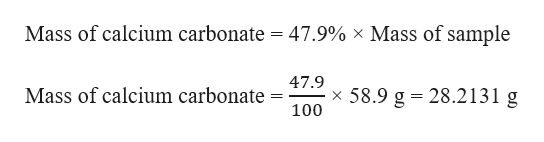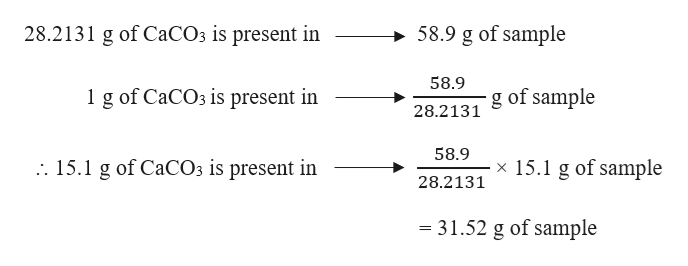# A sample is marked as containing 47.9 percent calcium carbonate by mass. How many grams of calcium carbonate are contained in 58.9 grams of the sample?How many grams of the sample above would contain 15.1 grams of calcium carbonate ?

Question
197 views

A sample is marked as containing 47.9 percent calcium carbonate by mass. How many grams of calcium carbonate are contained in 58.9 grams of the sample?

How many grams of the sample above would contain 15.1 grams of calcium carbonate ?

check_circle

Step 1

Given, mass of a sample = 58.9 g

The sample contains 47.9 percent calcium carbonate by mass.

The mass of calcium carbonate in the sample can be calculated as:help_outlineImage TranscriptioncloseMass of calcium carbonate = 47.9% x Mass of sample 47.9 X Mass of calcium carbonate 58.9 g 28.2131 g 100 fullscreen
Step 2

This means that 28.2131 g is present in 58.9 g of the sample.

Therefore, grams of the sample in 15.1 g of calcium carbo...help_outlineImage Transcriptionclose28.2131 g of C2CO3 is present in 58.9 g of sample 58.9 1 g of CaCO3 is present in 28.2131 of sample 58.9 x 15.1 g of sample . 15.1 g of CaCO3 is present in 28.2131 - 31.52 g of sample fullscreen

### Want to see the full answer?

See Solution

#### Want to see this answer and more?

Solutions are written by subject experts who are available 24/7. Questions are typically answered within 1 hour.*

See Solution
*Response times may vary by subject and question.
Tagged in

### General Chemistry